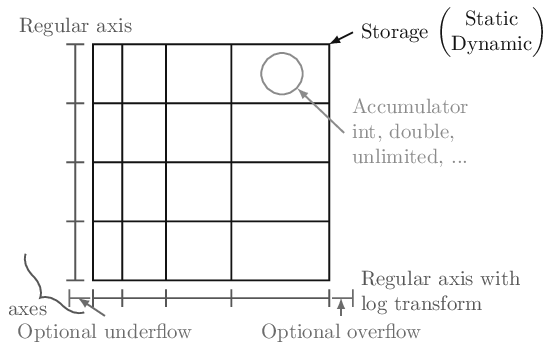# Quickstart#

All of the examples will assume the following import:

```import boost_histogram as bh
```

In boost-histogram, a histogram is collection of Axis objects and a storage.## Making a histogram#

You can make a histogram like this:

```hist = bh.Histogram(bh.axis.Regular(bins=10, start=0, stop=1))
```

If you’d like to type less, you can leave out the keywords:

```hist = bh.Histogram(bh.axis.Regular(10, 0, 1))
```

The exact same syntax is used for 1D, 2D, and ND histograms:

```hist3D = bh.Histogram(
bh.axis.Regular(10, 0, 100, circular=True),
bh.axis.Regular(10, 0.0, 10.0),
bh.axis.Variable([1, 2, 3, 4, 5, 5.5, 6]),
)
```

See Axes and Using Transforms.

You can also select a different storage with the `storage=` keyword argument; see Storages for details about the other storages.

## Filling a histogram#

Once you have a histogram, you can fill it using `.fill`. Ideally, you should give arrays, but single values work as well:

```hist = bh.Histogram(bh.axis.Regular(10, 0.0, 1.0))
hist.fill(0.9)
hist.fill([0.9, 0.3, 0.4])
```

## Slicing and rebinning#

You can slice into a histogram using bin coordinates or data coordinates using `bh.loc(v)`. You can also rebin with `bh.rebin(n)` or remove an entire axis using `sum` (technically as the third slice argument, though it is allowed by itself as well):

```hist = bh.Histogram(
bh.axis.Regular(10, 0, 1),
bh.axis.Regular(10, 0, 1),
bh.axis.Regular(10, 0, 1),
)
mini = hist[1:5, bh.loc(0.2) : bh.loc(0.9), sum]
# Will be 4 bins x 7 bins
```

See Indexing.

## Accessing the contents#

You can use `hist.values()` to get a NumPy array from any histogram. You can get the variances with `hist.variances()`, though if you fill an unweighted storage with weights, this will return None, as you no longer can compute the variances correctly (please use a weighted storage if you need to). You can also get the number of entries in a bin with `.counts()`; this will return counts even if your storage is a mean storage. See Plotting.

If you want access to the full underlying storage, `.view()` will return a NumPy array for simple storages or a RecArray-like wrapper for non-simple storages. Most methods offer an optional keyword argument that you can pass, `flow=True`, to enable the under and overflow bins (disabled by default).

```np_array = hist.view()
```

## Setting the contents#

You can set the contents directly as you would a NumPy array; you can set either values or arrays at a time:

```hist = 3.5
hist[bh.underflow] = 0  # set the underflow bin
hist2d[3:5, 2:4] = np.eye(2)  # set with array
```

For non-simple storages, you can add an extra dimension that matches the constructor arguments of that accumulator. For example, if you want to fill a Weight histogram with three values, you can dimension:

```hist[0:3] = [[1, 0.1], [2, 0.2], [3, 0.3]]
```

See Indexing.

## Accessing Axes#

The axes are directly available in the histogram, and you can access a variety of properties, such as the `edges` or the `centers`. All properties and methods are also available directly on the `axes` tuple:

```ax0 = hist.axes
X, Y = hist.axes.centers
```

See Axes.

## Saving Histograms#

You can save histograms using pickle:

```import pickle

with open("file.pkl", "wb") as f:
pickle.dump(h, f)

with open("file.pkl", "rb") as f:

assert h == h2
```

Special care was taken to ensure that this is fast and efficient. Please use the latest version of the Pickle protocol you feel comfortable using; you cannot use version 0, the version that was default on Python 2. The most recent versions provide performance benefits.

## Computing with Histograms#

As an complete example, let’s say you wanted to compute and plot the density:

```#!/usr/bin/env python3

from __future__ import annotations

import functools
import operator

import matplotlib.pyplot as plt
import numpy as np

import boost_histogram as bh

# Make a 2D histogram
hist = bh.Histogram(bh.axis.Regular(50, -3, 3), bh.axis.Regular(50, -3, 3))

# Fill with Gaussian random values
hist.fill(np.random.normal(size=1_000_000), np.random.normal(size=1_000_000))

# Compute the areas of each bin
areas = functools.reduce(operator.mul, hist.axes.widths)

# Compute the density
density = hist.view() / hist.sum() / areas

# Make the plot
fig, ax = plt.subplots()
mesh = ax.pcolormesh(*hist.axes.edges.T, density.T)
fig.colorbar(mesh)
plt.savefig("simple_density.png")
```## Comparing with Boost.Histogram#

This is built on the Boost.Histogram library.